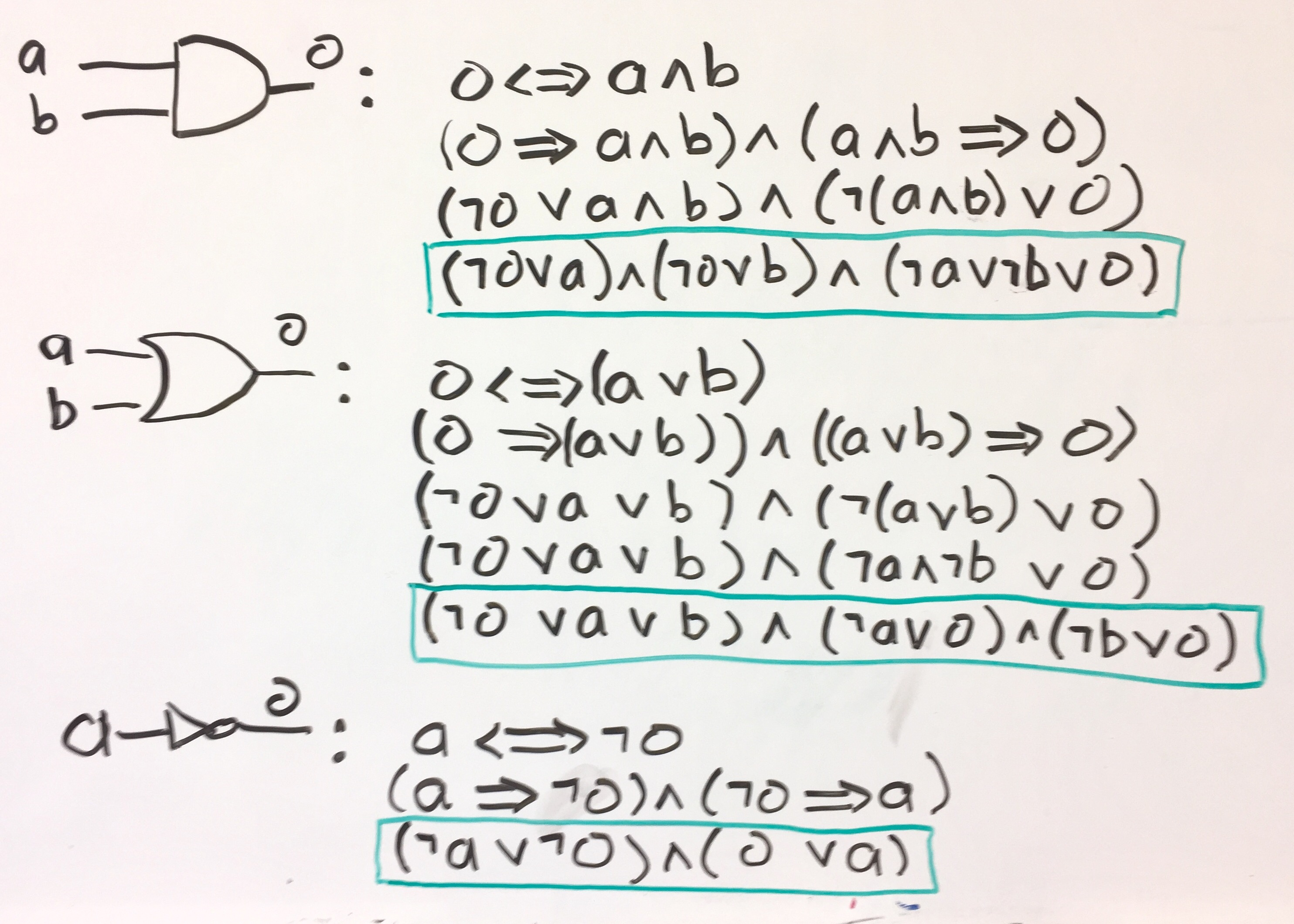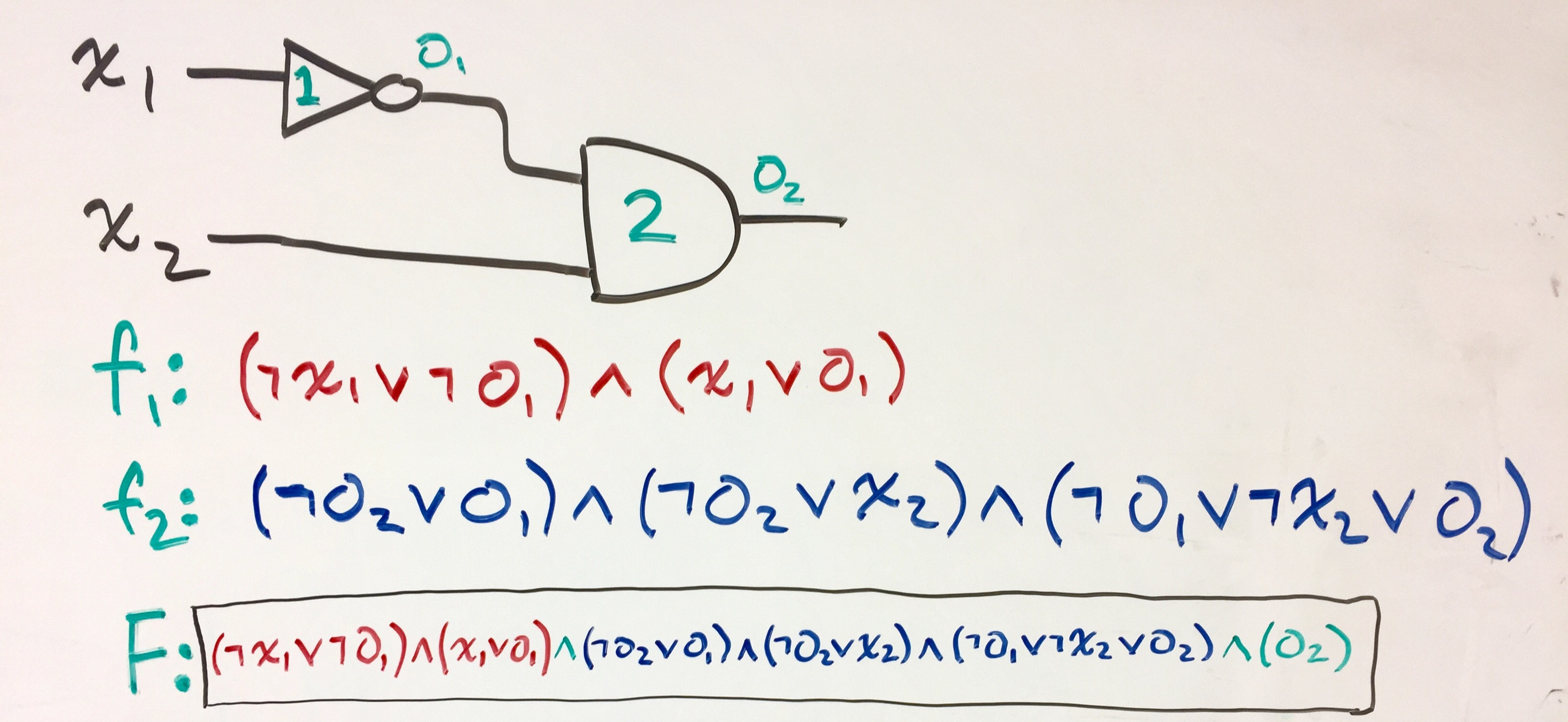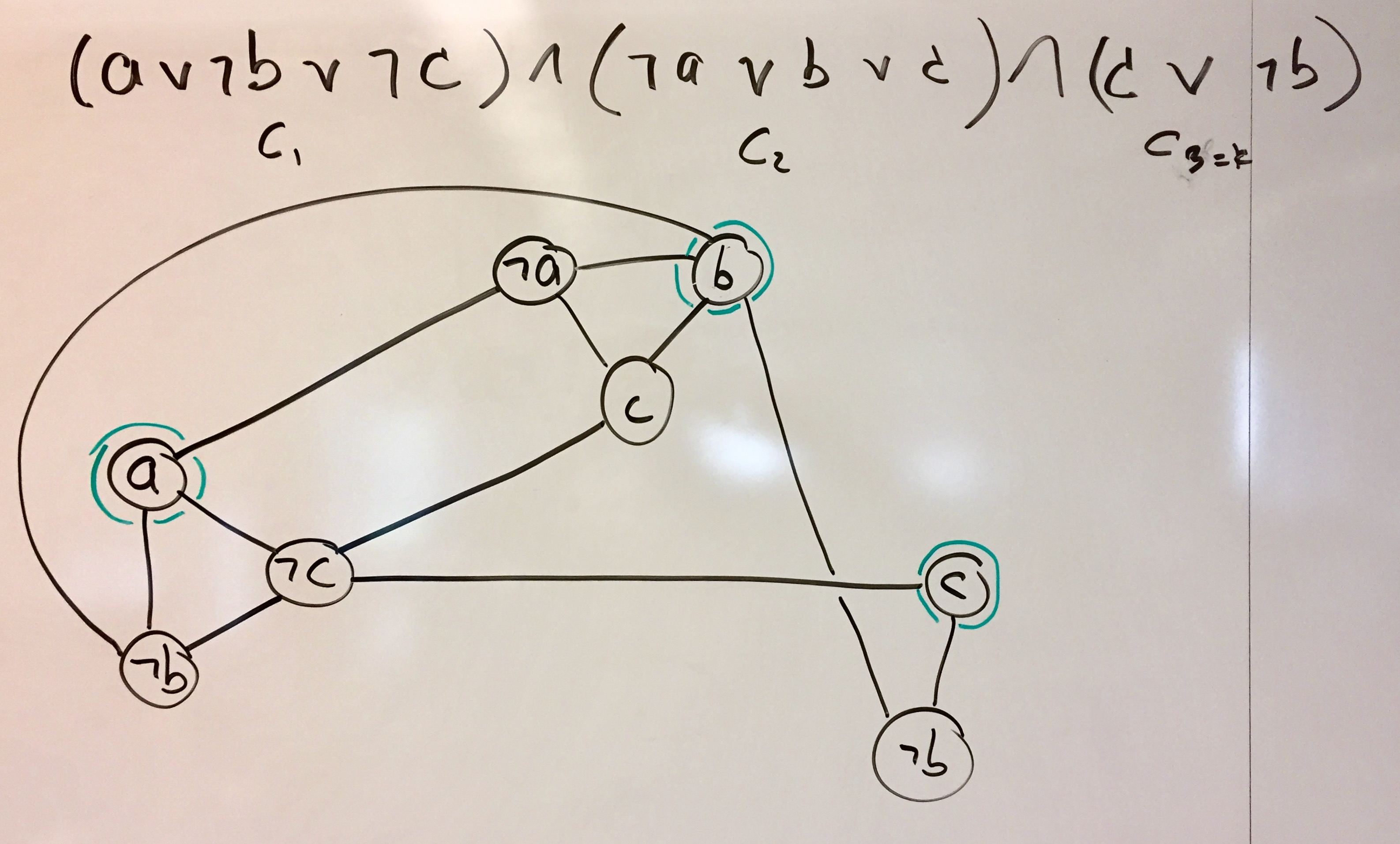These notes and Unit 6 sections 6 and 7.

## 3CNF-SAT

A propositional (boolean) formula $F$ is in Conjunctive Normal Form if it has the following form: $F = C_1 \wedge C_2 \wedge \cdots \wedge C_n$ where each $C_i$ is what's called a clause.

A clause is of the form $C_i = l_1 \vee l_2 \vee \cdots \vee c_k$ where each $l_j$ is what's called a literal.

A literal is simply a boolean variable, or its negation - i.e. $x_i$ or $\neg x_i$. Finially, a "3CNF" formula is a formula in CNF, with the added restriction that each clause has at most three literals.

So, for example the following is a 3CNF formula: $(a \vee \neg b \vee \neg c) \wedge (\neg a \vee b \vee c) \wedge (\neg a \vee \neg c)$

Of course, the 3CNF-SAT problem is simply this: given a formula in 3CNF, is there an assignment of values to the formula's variables for which the formula evaluates to true?

Formulas in CNF are really nice to work with, because they have such a simple, regular structure. So most logic applications require their input to be in CNF. 3CNF is even more restricted. That restriction is really nice for analysis purposes, as we'll see in the following.

## 3CNF-SAT is NP-Complete

In the below, we'll give a polynomial time reduction of CIRCUIT-SAT to 3CNF-SAT. First, we observe that we can take a circuit and expand it slightly (i.e. polynomially) to produce an equivalent circuit containing only AND, OR and NOT gates, and in which the AND and OR gates have only two inputs each. We discussed this in class. Now consider the following conversion of gate to 3CNF formula:With these rules in place, we can convert a circuit to its formula equivalent. Here's what we do:
1. number the gates $g_1,\ldots,g_k$ where the numbering is a topological sort. Note that this means that the last gate is the output for the whole circuit.
2. for each gate $g_i$ create a variable $o_i$, so now we have the original variables $x_1,\ldots,x_n$ plus the new $o_i$ variables, one per gate.
3. for each gate $g_i$ create formula $f_i$ using the above rules
4. return formula $f_1 \wedge f_2 \wedge \cdots \wedge f_k \wedge o_k$
The original circuit is satisfiable if and only if the formula is satisfiable (the proof is the same as the proof we gave for the conversion to SAT) and the process is clearly polynomial time. The idea behind the translation is that each $f_i$ encodes the assertion that the gate functions properly, for example that an AND-gate really outputs the "and" of its inputs. So the formula specifies that "gate 1 operates correctly, and cate 2 operates correctly, .... and gate $k$ operates correctly, and gate $k$ outputs TRUE".

Example:So, now that we have a polynomial time reduction from CIRCUIT-SAT to 3CNF-SAT, we've proved the following:

Theorem: 3CNF-SAT is NP-Complete.

## The INDEPENDENT-SET Problem

So far, all the problems that we've proven to be NP-Complete are closely-related problems ... all three are really about logic. Now let's consider a very different problem: a graph problem. The INDEPENDENT-SET Problem is this: Given unweighted, undirected graph $G$, and positive integer $k$, is there an independent set in $G$ of size $k$? Note: an independent set is a set of vertices from $G$ that have no edges between them.

## INDEPENDENT-SET is NP-Complete

Theorem: INDEPENDENT-SET is NP-Complete.

We prove this by giving a polynomial time reduction of 3CNF-SAT to INDEPENDENT-SET. So, we're given a 3CNF formula. Let $k$ be the number of clauses in the formua. We create a graph that has $k$ "clusters" of vertices, one cluster for each clause. A cluster for clause $C_i = (l_{i,1} \vee l_{i,2} \vee l_{i,3})$ consists of three vertices, each labeled with one of the three literals from the clause. If the clause only has 2 (or 1) literal, the cluster will only have 2 (or 1) vertices. Each vertex in a cluster is connected by an edge to every other vertex in the cluster. Also, a vertex in a given cluster labeled with literal $l$ has an edge to every vertex in the other clusters labeled $\neg l$. The claim is that this graph has an independent set of size $k$ if and only if the original formula is satisfiable.

Example: This example shows the graph derived from a 3CNF formula. The resulting INDEPENDENT-SET problem has $k=3$, since the formula has three clauses. In green, the vertices comprising an independent set of size 3 are circles. Notice that they correspond to a satisfying assignment of values to variables in formula.## NP-Complete Problems Surround Us!

For better or for worse, the world is full of NP-Complete problems. See Wikipedia's list of NP-Complete Problems. This includes problems like HAM-CYCLE, O/1-KNAPSACK, SIMPLE-KNAPSACK, HITTING-SET, PARTITION, and many, many more.